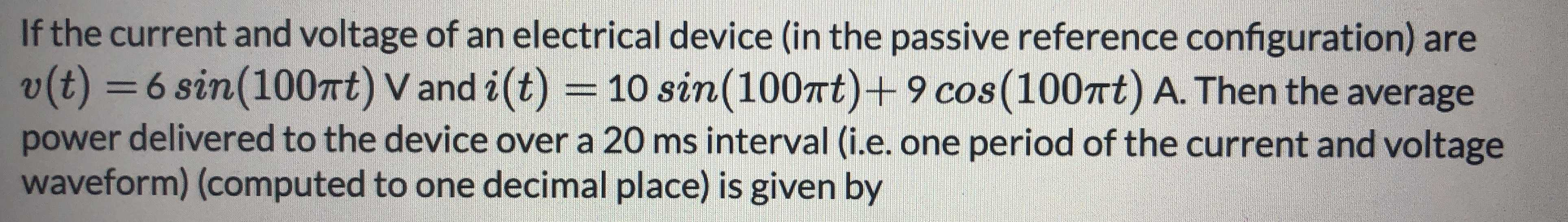### Still have math questions?

Algebra
QuestionIf the current and voltage of an electrical device (in the passive reference configuration) are

$$v ( t ) = 6 \sin ( 100 \pi t ) V$$ and $$i ( t ) = 10 \sin ( 100 \pi t ) + 9 \cos ( 100 \pi t ) A$$ . Then the average power delivered to the device over a $$20$$ ms interval (i.e. one period of the current and voltage waveform) (computed to one decimal place) is given by

$$\frac{2\pi}{100\pi}s= 0.02s= 20ms$$